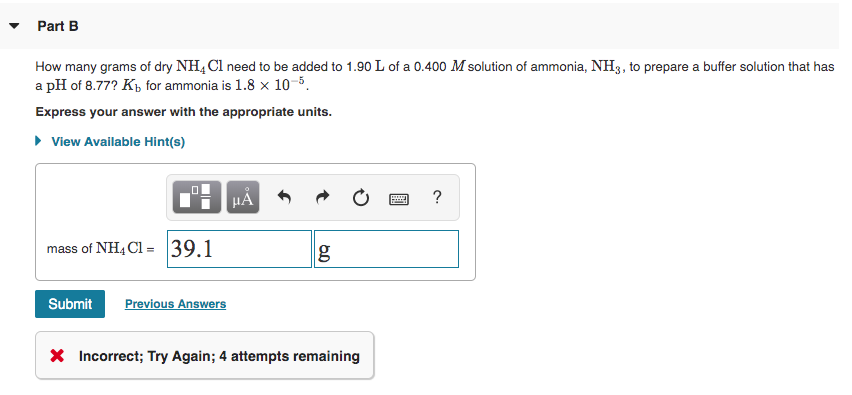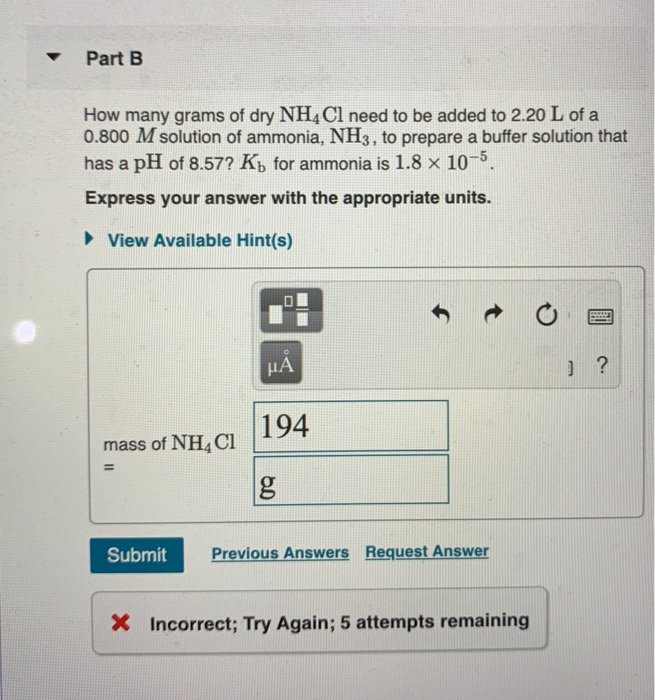Question

# How many grams of dry NH4Cl need to be added to 2.00 L of a 0.700...

How many grams of dry NH4Cl need to be added to 2.00 L of a 0.700 M solution of ammonia, NH3, to prepare a buffer solution that has a pH of 9.00? Kb for ammonia is 1.8×10−5. Express your answer with the appropriate units.

Kb = 1.8*10^-5

pKb = - log (Kb)

= - log(1.8*10^-5)

= 4.745

POH = 14 - pH

= 14 - 9

= 5

use formula for buffer

pOH = pKb + log ([NH4Cl]/[NH3])

5.0 = 4.7447 + log ([NH4Cl]/[NH3])

log ([NH4Cl]/[NH3]) = 0.2553

[NH4Cl]/[NH3] = 1.8

[NH4Cl]/0.7 = 1.8

[NH4Cl] = 1.26

volume , V = 2 L

use:

number of mol,

n = Molarity * Volume

= 1.26*2

= 2.52 mol

Molar mass of NH4Cl,

MM = 1*MM(N) + 4*MM(H) + 1*MM(Cl)

= 1*14.01 + 4*1.008 + 1*35.45

= 53.492 g/mol

use:

mass of NH4Cl,

m = number of mol * molar mass

= 2.52 mol * 53.49 g/mol

= 1.348*10^2 g

#### Earn Coins

Coins can be redeemed for fabulous gifts.

Similar Homework Help Questions
• ### How many grams of dry NH4Cl need to be added to 1.50 L of a 0.700...

How many grams of dry NH4Cl need to be added to 1.50 L of a 0.700 M solution of ammonia, NH3, to prepare a buffer solution that has a pH of 8.56? Kb for ammonia is 1.8×10−5.

• ### How many grams of dry NH4Cl need to be added to 1.90 L of a 0.800...

How many grams of dry NH4Cl need to be added to 1.90 L of a 0.800 M solution of ammonia, NH3, to prepare a buffer solution that has a pH of 8.74? Kb for ammonia is 1.8×10^−5. Express your answer with the appropriate units.

• ### 4B. How many grams of dry NH4Cl need to be added to 1.80 L of a...

4B. How many grams of dry NH4Cl need to be added to 1.80 L of a 0.100 M solution of ammonia, NH3, to prepare a buffer solution that has a pH of 8.93? Kb for ammonia is 1.8×10^−5. Express your answer with the appropriate units.

• ### How many grams of dry NH4Cl need to be added to 2.10 L of a 0.500 M solution of ammonia, NH3, to prepare a buffer solu...

How many grams of dry NH4Cl need to be added to 2.10 L of a 0.500 M solution of ammonia, NH3, to prepare a buffer solution that has a pH of 8.76? Kb for ammonia is 1.8×10−5. Express your answer with the appropriate units.

• ### How many grams of dry NH4Cl need to be added to 1.90 L of a 0.800...

How many grams of dry NH4Cl need to be added to 1.90 L of a 0.800 M solution of ammonia, NH3, to prepare a buffer solution that has a pH of 8.74? Kb for ammonia is 1.8×10^-5.

• ### Part B How many grams of dry NH4Cl need to be added to 1.90 L of...Part B How many grams of dry NH4Cl need to be added to 1.90 L of a 0.400 M solution of ammonia, NH3, to prepare a buffer solution that has a pH of 8.77? Kb for ammonia is 1.8 x 10-5 Express your answer with the appropriate units. View Available Hint(s) CHÅN O a? mass of NH4C1 = 39.1 g Submit Previous Answers X Incorrect; Try Again; 4 attempts remaining

• ### How many grams of dry NH4Cl need to be added to 2.30 L of a 0.100 M solution of ammonia,NH3, to prepare a buffer soluti...

How many grams of dry NH4Cl need to be added to 2.30 L of a 0.100 M solution of ammonia,NH3, to prepare a buffer solution that has a pH of 9.00? Kb for ammonia is 1.8x 10^-5.

• ### - Part B How many grams of dry NH4Cl need to be added to 2.20 L...- Part B How many grams of dry NH4Cl need to be added to 2.20 L of a 0.800 M solution of ammonia, NH3, to prepare a buffer solution that has a pH of 8.57? Ký for ammonia is 1.8 x 10-5. Express your answer with the appropriate units. View Available Hint(s) UA 194 mass of NHACI Submit Previous Answers Request Answer X Incorrect; Try Again; 5 attempts remaining

• ### How many grams of dry NH4Cl need to be added to 2.50 L of a 0.500 M solution of ammonia, NH3, to prepare a buffer soluti...

How many grams of dry NH4Cl need to be added to 2.50 L of a 0.500 M solution of ammonia, NH3, to prepare a buffer solution that has a pH of 8.74? Kb for ammonia is 1.8*10^-5.

• ### How many grams of dry NH4Cl need to be added to 1.70 L of a 0.100 M solution of ammonia, NH3, to prepare a buffer solu...

How many grams of dry NH4Cl need to be added to 1.70 L of a 0.100 M solution of ammonia, NH3, to prepare a buffer solution that has a pH of 8.62? Kb for ammonia is 1.8×10−5.